# Confidence intervals continued

04/12/2021

## Instructions:

Use the left and right arrow keys to navigate the presentation forward and backward respectively. You can also use the arrows at the bottom right of the screen to navigate with a mouse.

FAIR USE ACT DISCLAIMER:
This site is for educational purposes only. This website may contain copyrighted material, the use of which has not been specifically authorized by the copyright holders. The material is made available on this website as a way to advance teaching, and copyright-protected materials are used to the extent necessary to make this class function in a distance learning environment. The Fair Use Copyright Disclaimer is under section 107 of the Copyright Act of 1976, allowance is made for “fair use” for purposes such as criticism, comment, news reporting, teaching, scholarship, education and research.

## Outline

• The following topics will be covered in this lecture:

• A review of confidence intervals
• Estimating the necessary sample size for an error tolerance
• One-sided confidence bounds
• Confidence intervals for the mean, with unknown population standard deviation
• Large sample size
• Student t distribution
• Student t confidence intervals

### Introduction to confidence intervals – continued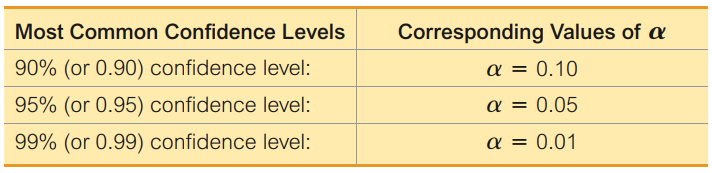Courtesy of Mario Triola, Essentials of Statistics, 6th edition

• Let’s take an example confidence level of $$95\%$$ – this corresponds to a rate of failure of $$5\%$$ over infinitely many replications.
• Generally, we will write the confidence level as, $(1 - \alpha) \times 100\%$ so that we can associate this confidence level with its rate of failure $$\alpha$$.
• In our problem, because $$Z = \frac{\overline{X} - \mu }{\frac{\sigma}{\sqrt{n}}}$$ has a standard normal distribution, we may write \begin{align} P\left(-z_\frac{\alpha}{2} \leq \frac{\overline{X} - \mu }{\frac{\sigma}{\sqrt{n}}} \leq z_\frac{\alpha}{2}\right) = 1 - \alpha \end{align} where
• we want to find the critical value $$z_\frac{\alpha}{2}$$ for which:
• $$(1-\frac{\alpha}{2})\times 100\%$$ of the area under the normal density lies to the left of $$z_\frac{\alpha}{2}$$; and
• $$(1-\frac{\alpha}{2})\times 100\%$$ of the area under the normal density lies to the right of $$-z_\frac{\alpha}{2}$$.
• Put together, $$(1-\alpha)\times 100\%$$ of values lie within $$[-z_\frac{\alpha}{2},z_\frac{\alpha}{2}]$$ in the standard normal.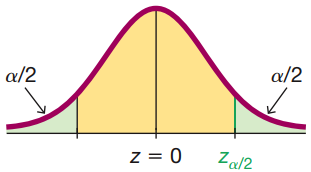### Introduction to confidence intervals – continued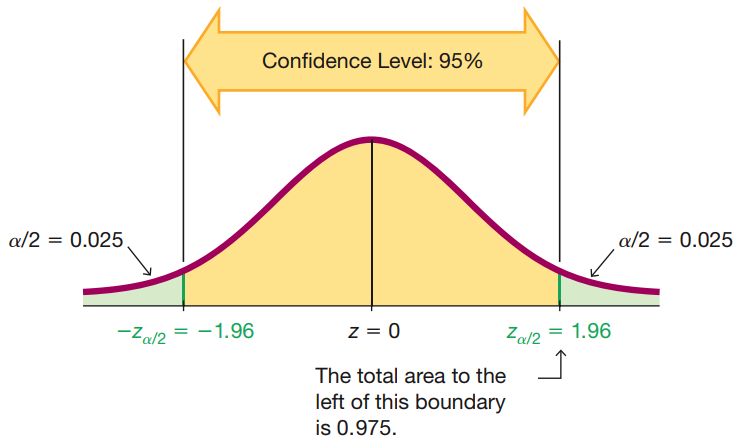Courtesy of Mario Triola, Essentials of Statistics, 6th edition

• In the figure to the left, we see exactly thus how to find the width of the region for a given confidence level.
• For a given confidence level $$(1 -\alpha)\times 100\%$$, we will find the particular $$\alpha$$.
• We then find the associated $$z_\frac{\alpha}{2}$$ critical value.
• This critical value is associated to the measure of extremeness of finding an observation that lies far away from the mean.
• Particularly, only $$\alpha \times 100\%$$ of the population lies outside of the region $$[-z_\frac{\alpha}{2},z_\frac{\alpha}{2}]$$ in the standard normal.
• Manipulating the earlier probability statement, we find that \begin{align} & P\left(-z_\frac{\alpha}{2} \leq \frac{\overline{X} - \mu }{\frac{\sigma}{\sqrt{n}}} \leq z_\frac{\alpha}{2}\right) = 1 - \alpha\\ \Leftrightarrow & P\left( \overline{X} - \frac{\sigma}{\sqrt{n}}z_\frac{\alpha}{2} \leq \mu \leq \overline{X} + \frac{\sigma}{\sqrt{n}} z_\frac{\alpha}{2}\right) = 1 - \alpha. \end{align}
• The above represents the traditional way to construct a confidence interval; with $$(1-\alpha)\times 100\%$$ confidence $L \leq \mu \leq U \Leftrightarrow \overline{X} - \frac{\sigma}{\sqrt{n}}z_\frac{\alpha}{2} \leq \mu \leq \overline{X} + \frac{\sigma}{\sqrt{n}} z_\frac{\alpha}{2}$

### Introduction to confidence intervals – continued

• Note, the last argument only works for a normal population.
• However, we get a very good approximation with $$n$$ large enough for non-normal populations.
• The last argument also required that the variance $$\sigma^2$$ was known.

• If $$\sigma^2$$ is unknown, we need to change the argument slightly, which is the reason we belabored z-scores in this argument.
• To compute the confidence intervals as above, we need to introduce a new function, the quantile function:

• qnorm(p, mean, sd) – this is the quantile function that gives the critical value associated to the value $$\alpha=p$$.
• The quantile function returns the value $$z_p$$ for which $$p\times 100 \%$$ of the area under the standard normal lies to the left of $$z_p$$ and $$(1-p)\times 100\%$$ lies to the right.
• Compared to our earlier example:
qnorm(0.025)

 -1.959964

qnorm(0.975)

 1.959964

• By the symmetry of the normal, we can thus compute $$z_\frac{\alpha}{2}$$=qnorm(p) where $$p=1-\frac{\alpha}{2}$$.

### Introduction to confidence intervals – continued

• Suppose we know that, $$\overline{X}$$ is the sample mean from a normal population with (unknown) mean $$\mu= 10$$, standard deviation $$\sigma= 2$$ and sample size $$n=16$$, then

$\overline{X} \sim N\left(10, \frac{4}{16}\right).$

• Notice that the standard error is thus given as $$\sigma_\overline{X} = \sqrt{\frac{1}{4}} = \frac{1}{2}$$

• If $$\overline{X}$$ is observed to take the value $$\overline{x}=9$$, then we can construct the $$95\%$$ confidence interval for $$\mu$$ as,

se <- 0.5
z_alpha_over_2 <- qnorm(0.975)
ci <- c(9 - se*z_alpha_over_2 , 9+se*z_alpha_over_2)
ci

 8.020018 9.979982

• Notice, the above confidence interval does not contain the true population mean $$\mu=10$$…

### Introduction to confidence intervals – continued

• Let's suppose instead we repeat the argument but select $$\alpha=0.01$$.

• This corresponds to a critical value $$z_\frac{\alpha}{2}=z_{0.005}$$.

• To compute the the corresponding critical value, we are looking for p=$$1-\frac{\alpha}{2}=0.995$$

se <- 0.5
z_alpha_over_2 <- qnorm(0.995)
ci <- c(9 - se*z_alpha_over_2 , 9+se*z_alpha_over_2)
ci

  7.712085 10.287915

• Notice that this $$(1-\alpha)\times 100\% = 99\%$$ confidence interval does contain the true mean.

• This confidence interval is somewhat wider as we want to guarantee that the procedure will work $$99\%$$ of the time.

• Therefore, we need to include a wider range of plausible values when we construct such an interval.

### Introduction to confidence intervals – continued

• What we are imagining when we construct confidence intervals is the following.
• Based on some particular sample $$X_{j,1},\cdots, X_{j,n}$$ of size $$n$$ indexed by $$j$$, we will get some particular value for the confidence interval.
• If we replicate the sample of size $$n$$, indexed by $$j$$, we will almost surely find a new confidence interval based on each replicate.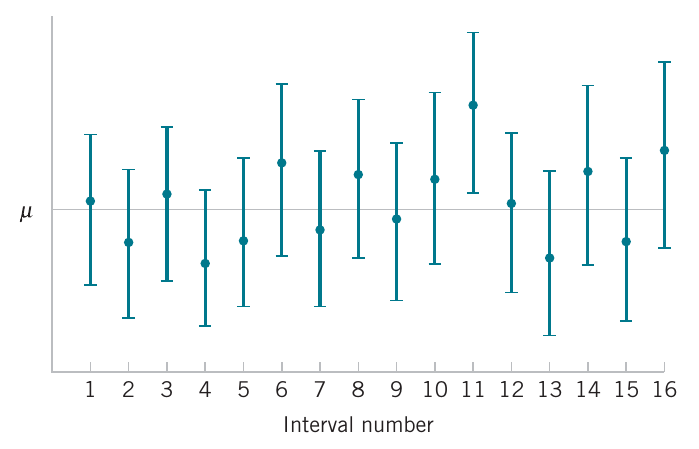Courtesy of Montgomery & Runger, Applied Statistics and Probability for Engineers, 7th edition

• Our goal in constructing a confidence interval is thus to catch the true parameter value with the confidence level $$(1-\alpha)\times 100\%$$ out of all replicates.
• If we want higher confidence, we need wider intervals to catch the true value at a higher rate.
• However, the normal confidence interval, $\overline{X} - \frac{\sigma}{\sqrt{n}}z_\frac{\alpha}{2} \leq \mu \leq \overline{X} + \frac{\sigma}{\sqrt{n}} z_\frac{\alpha}{2}$ also has a width that depends on the sample size.
• This is of course, as we discussed in the central limit theorem, the precision of the sample mean $$\overline{X}$$ increases for larger sample sizes, with a standard deviation that shrinks like $$\frac{1}{\sqrt{n}}$$.
• This allows us to select a sample size for a target precision, given a level of confidence.

## Choosing the sample size

• In situations whose sample size can be controlled, we can choose $$n$$ so that we are $$(1 − \alpha)\times 100\%$$ confident that the error in estimating $$\mu$$ is less than a specified bound on the error $$E$$.
• The appropriate sample size is found by choosing $$n$$ such that $z_\frac{\alpha}{2} \hat{\sigma}_\overline{X} = z_\frac{\alpha}{2} \frac{\sigma}{\sqrt{n}} = E.$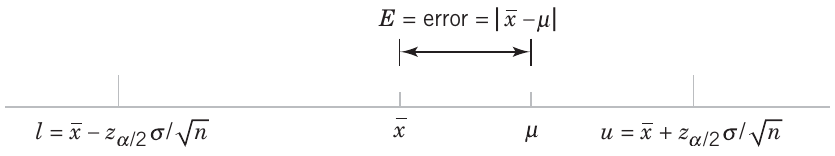Courtesy of Montgomery & Runger, Applied Statistics and Probability for Engineers, 7th edition

• Notice, $$n$$ does not affect the choice of $$z_\frac{\alpha}{2}$$ which is based on the desired confidence level;
• rather, this changes the size of the standard error, which decreases proportionally to $$\frac{1}{\sqrt{n}}$$.
• Solving this equation gives the following formula for $$n$$: \begin{align} &E = z_\frac{\alpha}{2} \frac{\sigma}{\sqrt{n}} \\ \Leftrightarrow & \frac{1}{\sqrt{n}} = \frac{E}{z_\frac{\alpha}{2} \sigma} \\ \Leftrightarrow & n = \left(\frac{z_\frac{\alpha}{2} \sigma}{E}\right)^2 \end{align}
• For a specified error tolerance $$E$$, we can thus choose $$n$$ such that $$\mu$$ lies within $$E = z_\frac{\alpha}{2} \frac{\sigma}{\sqrt{n}}$$ of the sample mean $$\overline{X}$$ with $$(1-\alpha)\times 100 \%$$ confidence.
• $$E$$ is a chosen radius of the confidence interval around the point estimate, in which we want to “catch” the true value.

### Choosing the sample size – continued

• Putting this formally:
Sample Size for Specified Error on the Mean, Variance Known
If $$\overline{X}$$ is used as an estimate of $$\mu$$, we can be $$(1 − \alpha)\times 100\%$$ confident that the error $$\vert \overline{x} - \mu\vert$$ will not exceed a specified amount $$E$$ when the sample size is $n = \left(\frac{z_\frac{\alpha}{2} \sigma}{E}\right)^2.$
• Note that the above was directly a consequence of the central limit theorem, and the fact that

$\overline{X} \sim N\left(\mu, \frac{\sigma^2}{n}\right).$

when the underlying population is normal (or approximately for large $$n$$ for non-normal populations).

### Choosing the sample size – example

• Suppose that we want to determine how many specimens must be tested to ensure that the $$95\%$$ CI on $$\mu$$ for a normal population with known standard deviation $$\sigma=1$$ falls below some specified error tolerance.

• For a $$95\%$$ confidence interval, we have $$\alpha=1-0.95=0.05$$.

• We therefore require that $$1-\frac{\alpha}{2} = 1-0.025=0.975$$ of the area lies to the left and $$\frac{\alpha}{2}=0.025$$ lies to the right of $$z_\frac{\alpha}{2}$$.

• If $$E=10\%$$, we would write,

\begin{align} n &= \left(\frac{z_\frac{\alpha}{2} \sigma}{E}\right)^2\\ &= \left(\frac{z_{0.025} \times 1}{0.10}\right)^2 \end{align}

• In R we can write

E <- 0.1
sigma <- 1.0
z_alpha_over_2 <- qnorm(0.975)
n_ten_percent <- ( (z_alpha_over_2 * sigma) / E )^2
n_ten_percent

 384.1459


### Choosing the sample size – example

• Notice, with our last choice of a $$10\%$$ error tolerance we had a corresponding sample size of
n_ten_percent

 384.1459

• Suppose we require this estimate to be more precise, say $$E=5\%$$ or $$E=1\%$$.

• We can revise our previous estimate by

E <- 0.05
n_five_percent <- ( (z_alpha_over_2 * sigma) / E )^2
n_five_percent

 1536.584

• and
E <- 0.01
n_one_percent <- ( (z_alpha_over_2 * sigma) / E )^2
n_one_percent

 38414.59


### Choosing the sample size – example

• In the last example, we saw the necessary sample size grow as follows:
x_vals <- c(0.01, 0.05, 0.10)
y_vals <- c(n_one_percent, n_five_percent, n_ten_percent)
par(cex = 2.0, mar = c(5, 4, 4, 2) + 0.3)
plot(x_vals, y_vals, xlab = "Error tolerance", ylab="Necessary sample size", type="b")